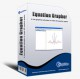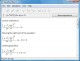Equation Grapher 2.2 Equation grapher is a free graphing calculator to graph equations. FreewareQ Graph 1.0 Graph any explicit or implicit relation or inequality in rectangular or polar coordinates. FreewareLiveMath Viewer 3.5.5 LiveMath Viewer is the demo version of LiveMath Maker. FreewareEquation Wizard 1.21 Automatically solves algebraic equations of any order written in any form SharewareGraph550 0.5 Graph550 is a program to graph mathematical equations. FreewareNA_WorkSheet 2006-02-22 NA_WorkSheet project is a collective aggregation of algorithms written in Java that implements various numerical analysis solutions and techniques. GPLPDF Equation 0.7 PDF Equation gives you quick access to LaTeX's typesetting power in almost every Mac OS X application. Freeware3D Grapher 1.21 Plot and animate 2D and 3D equation and table-based graphs with ease SharewareEquation Service 1.0 Equation Service is a program that uses pdflatex to produce small PDF files containing equations and other text. FreewareChemical Equation Expert 2.12a Chemical Equation Expert is an integrated tool for chemistry professionals Freeware